GeeksforGeeks App
Open AppBrowser
Continue

## Related Articles

• RD Sharma Class 10 Solutions

# Class 10 RD Sharma Solutions – Chapter 14 Coordinate Geometry – Exercise 14.2 | Set 3

### Question 40. Prove that the points (3, 0), (4, 5), (-1, 4), and (-2, -1) taken in order, form a rhombus. Also, find its area.

Solution:

Let us considered the given points are A(3, 0), B(4, 5), C(-1, 4) and D(-2, -1)

Now we find the length of the sides and diagonals,

By using distance formula

So, AB =AB2 = (4 + 3)2 + (5 – 0)

= (1)2 + (5)2

= 1 + 25 = 26

Similarly, BC2 = (-1 – 4)2 + (4 – 5)2

= (-5)2 + (-1)2 = 25 + 1 = 26

CD2 = (-2 + 1)2 + (-1 – 4)2

= (-1)2 + (-5)2 = 1 + 25 = 26

and DA2 = (3 + 2)2 + (0 + 1)

= (5)2 + (1)2 = 25 + 1 = 26

Diagonal AC2 = (-1 – 3)2 + (4 – 0)2

= (-4)2 + (4)2 = 16 + 16 = 32

and BD2 = (-2 – 4)2 + (-1 – 5)2

= (-6)2 + (-6)2 = 36 + 36 = 72

So, we conclude that the sides AB = BC = CD = DA and diagonal AC is not equal to BD

Hence, ABCD is a rhombus

Now we find the area of rhombus ABCD = Product of diagonals/2

= (√32 × √72)/2

= (√16 × 2 × 2 × 36)/2

= 4 × 2 × 6/2

= 24 sq. units

### Question 41. In the seating arrangement of desks in a classroom three students Rohini, Sandhya, and Bina are seated at A (3, 1), B (6, 4), and C (8, 6). Do you think they are seated in a line?

Solution:

Given that A (3, 1), B (6, 4) and C (8, 6)

Now we find the length of the sides and diagonals,

By using distance formula

AB =AB2 = (6 – 3)2 + (4 – 1)2

= (3)2 + (3)2 = 9 + 9 = 18

Similarly, BC2 = (8 – 6)2 + (6 – 4)2

= (2)2 + (2)2 = 4 + 4 = 8

and BC2 = (8 – 6)2 + (6 – 4)2

= (2)2 + (2)2 = 4 + 4 = 8

and CA2 = (3 – 8)2 + (1 – 6)2

= (-5)2 + (-5)2 = 25 + 25 = 50

AB = √18 = √9 * 2 = 3√2

BC = √8 = √4 * 2 = 2√2

and CA = √50 = √25 * 2 = 5√2

AB + BC = 3√2 + 2√2 = 5√2 = CA

Hence, A, B and C are collinear points. Hence, they are seated in a line.

### Question 42. Find a point on y-axis which is equidistant from the points (5, -2) and (-3, 2).

Solution:

Let us assume P be the point lies on y-axis. So, its x = 0, so the coordinates of P is (0, y)

It is given that the point P (0, y) is equidistant from the points A(5, -2) and B(-3, 2).

So, PA = PB

Also, PA2 = PB2

Now by using distance formula, we get

(5 – 0)2 + (-2 – y)2 = (-3 – 0)2 + (2 – y)2

25 + 4 + y2 + 4y = 9 + 4 – 4y + y2

y2 + 4y + 4y – y2 = 13 – 29

8y = -16

y = -16/8 = 2

Hence, the required point P is (0,-2)

### Question 43. Find a relation between x and y such that the point (x, y) is equidistant from the points (3, 6) and (-3, 4).

Solution:

Let us considered P (x, y) is equidistant from A(3, 6) and B(-3, 4)

So, PA = PB

Also, PA2 = PB2

Now by using distance formula, we getOn squaring both sides, we get

(x – 3)2 + (y – 6)2 = (x + 3)2 + (y – 4)2

x2 – 6x + 9 + y2 – 12y + 36 = x2 + 6x + 9 = y2 – 8y + 16

-6x – 12y + 45 = 6x – 8y + 25

-6x – 6x – 12y + 8y + 45 – 25 = 0

-12 – 4y + 20 = 0

3x + y – 5 = 0

3x + y = 5

### Question 44. If a point A (0, 2) is equidistant from the points B (3, p) and C (p, 5), then find the value of p.

Solution:

Given that the point A (0, 2) is equidistant from the points B (3, p) and C (p, 5)

Now by using distance formula, we getIt is given that AB = AC

√p2 – 4p + 13 = √p2 + 9

So, on squaring both side, we get

= p2 – 4p + 13 = p2 + 9

p2 – 4p – p2 = 9 – 13

-4p = -4

p = 1

Hence, the value of p is 1

### Question 45. Prove that the points (7, 10), (-2, 5), and (3, -4) are the vertices of an isosceles right triangle.

Solution:

Let us considered the points are A (7, 10), B (-2, 5) and C (3, -4)

Now we find the length of the sides

By using distance formula

Now AB =Similarly, BC =and AC =So, we conclude that AB = BC = √106 and AB2 + BC2 = AC2

Hence, ABC is an isosceles right triangle

### Question 46. If the point P (x, 3) is equidistant from the points A (7, -1) and B (6, 8), find the value of x and  the distance AP.

Solution:

It is given that Point P (x, 3) is equidistant from the points A (7, -1) and B (6, 8)

So, PA = PBOn squaring both sides, we get

(x – 7)2 + (4)2 = (x – 6)2 + (-5)2

x2 – 14x + 49 + 16 = x2 – 12x + 36 + 25

x2 – 14x + 65 = x2 – 12x + 61

x2 – 14x + 12x – x2 = 61 – 65

-2x = -4

x = -4/-2 = 2

x = 2

Now we find the distance### Question 47. If A (3, y) is equidistant from points P (8, -3) and Q (7, 6), find the value of y and find the distance AQ.

Solution:

It is given that point A (3, y) is equidistant from P (8, -3) and Q (7, 6)

So, AP = AQOn squaring both sides, we get

(3 – 8)2 + (y + 3)2 = (-4)2 + (y – 6)2

(-5)2 + y2 + 6y + 9 = 16 + y2 – 12y + 36

25 + y2 + 6y + 9 = 16 + y2 – 12y + 36

y2 + 6y – y2 + 12y = 36 – 9 – 25 + 16

18y = 18

y = 18/18 = 1

y = 1

Now we find the distance### Question 48. If (0, -3) and (0, 3) are the two vertices of an equilateral triangle, find the coordinates of its third vertex.

Solution: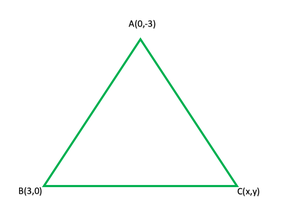Given that the A (0, -3) and B (0, 3) are the two vertices of an equilateral triangle ABC

Let us assume that the coordinates of the third vertex be C (x, y)

In equilateral triangle, AC = AB

So,

(x – 0)2 + (y + 3)2 = (0 – 0)2 + (3 + 3)2

x2 + (y + 3)2 = 0 + (6)2 = 36

x2 + y2 + 6y + 9 = 36

x2 + y2 + 6y = 36 – 9 = 27              …….(i)

Also, BC = AB

(x – 0)2 + (y – 3)2 = 36

x2 + y2 + 9 – 6y = 36

x2 + y2 – 6y = 36 – 9 = 27              ……..(ii)

So, from eq (i) and (ii), we get

x2 + y2 + 6y = x2 + y2 – 6y

x2 + y2 + 6y – x2 – y2 + 6y = 0

12y = 0

y = 0

Now put the value of y in eq(i)

x2 + y2 + 6y = 27

x2 + 0 + 0 = 27

x = ±√27 = ±3√3

So, the coordinates of third point is(3√3, 0) or (-3√3, 0)

### Question 49. If the point P (2, 2) is equidistant from the points A (-2, k) and B (-2k, -3), find k. Also, find the length of AP.

Solution:

Given that the point P (2, 2) is equidistant from the points A (-2, k) and B (-2k, -3)

So, AP = BP

(2 + 2)2 + (2 – k)2 = (2 + 2k)2 + (2 + 3)2

(4)2 + (2 – k)2 = (2 + 2k)2 + (5)2

16 + 4 + k2 – 4k = 4 + 4k2 + 8k + 25

4k2 + 8k + 29 – 16 – 4 – k2 + 4k = 0

3k2 + 12k + 9 = 0

k2 + 4k + 3 = 0

k2 + k + 3k + 3 = 0

k(k + 1) + 3(k + 1) = 0

(k + 1)(k + 3) = 0

So, the value of k either k + 1 = 0, then k = -1

or k + 3 = 0, then k = -3

Therefore, k = -1, -3

Now we find the distance### Question 50. Show that ∆ABC, where A (-2, 0), B (2, 0), C (0, 2) and ∆PQR, where P (-4, 0), Q (4, 0), R (0, 4) are similar.

Solution:

Given that In ∆ABC, the vertices are A (-2, 0), B (2, 0), C (0, 2)

In ∆PQR, the vertices are P (-4, 0), Q (4, 0), R (0, 4)

Show that ∆ABC ~ ∆PQR

So,Now,So, AB/PQ = 4/8 = 1/2

BC/QR = 2√2/4√2  = 1/2

CA/PQ = 2√2/4√2 = 1/2

So, AB/PQ = BC/QR = CA/RP

By using SSS

∆ABC ~ ∆PQR

### Question 51. An equilateral triangle has two vertices at the points (3, 4), and (-2, 3). Find the coordinates of the third vertex.

Solution: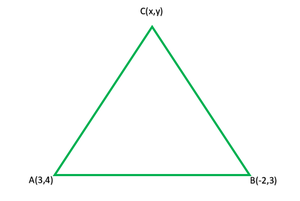Given that the A (3, 4) and B (-2, 3) are the two vertices of an equilateral triangle ABC

Let us assume that the coordinates of the third vertex be C (x, y)

NowAs we know that in equilateral triangle, AB = BC = CA

So, BC = ABOn squaring both side we get

(x + 2)2 + (y – 3)2 = 26

x2 + 4x + 4 + y2 – 6y + 9 = 26

x2 + y2 + 4x – 6y + 13 = 26

x2 + y2 + 4x – 6y = 26 – 13 = 12     ………..(i)

Similarly, CA = ABOn squaring both side we get

(3 – x)2 + (4 – y)2 = 26

9 + x2 – 6x + 16 + y2 – 8y = 26

x2 + y2 – 6x – 8y + 25 = 26

x2 + y2 – 6x – 8y = 26 – 25 = 1             …….(ii)

Now on subtracting eq(ii) from (i), we get

10x + 2y = 12

5x + y = 6                        ……..(iii)

y = 6 – 5x

Now substituting the value of y in eq(i), we get

x2 + (6 – 5x)2 + 4x – 6(6 – 5x) = 13

x2 + 36 + 25x2 – 60x + 4x – 36 +  30x – 13 = 0

26x2 – 26x – 13 = 0

2x2 – 2x – 1 = 0

Here, a = 2, b = -2, c = -1When x = (1 + √3)/2, then y = 6 – 5x = 6 – 5((1 + √3)/2) = (7 – 5√3)/2

Or when x = (1 – √3)/2, then y = 6 – 5x = 6 – 5((1 – √3)/2) = (7 + 5√3)/2

Hence, the co-ordinates of the point C is ((1 + √3)/2, (7 – 5√3)/2) or ((1 – √3)/2, (7 + 5√3)/2)

### Question 52. Find the circumcenter of the triangle whose vertices are (-2, -3), (-1, 0), (7, -6).

Solution: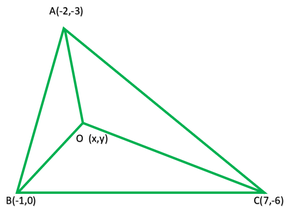Given that the vertices of ∆ABC are A(-2, -3), B(-1, 0), and C(7, -6), and

let us assume that O is the circumcenter ∆ABC. So, the coordinates of O will be (x, y)

So, OA = OB = OC

Or OA2 = OB2 = OC2

NowOA2 = (x + 2)2 + (y + 3)2

= x2 + 4x + 4 + y2 + 6y + 9

= x2 + y2 + 4x + 6y + 13

OB2 = (x + 1)2 + (y + 0)2

= x2 + 2x + 1 + y2

= x2 + y2 + 2x + 1

OC2 = (x – 7)2 + (y + 6)2

= x2 – 14x + 49 + y2 + 12y + 36

= x2 + y2 – 14x + 12y + 85

OA2 = OB2

x2 + y2 + 4x + 6y + 13 = x2 + y2 + 2x + 1

4x + 6y -2y = 1 – 13

2x + 6y = -12

x + 3y = -6       ………(i)

OB2 = OC2

x2 + y2 + 2x + 1 = x2 + y2 – 14x + 12y + 85

2x + 14x – 2y = 85 – 1

16x – 12y = 84

4x – 3y = 21              ………(ii)

From eq (i), we get

x = -3y – 6

On substituting the value of x in eq (ii)

4(-3y – 6) – 3y = 21

-12 – 24 – 3y = 21

-15y = 21 + 24

-15y = 45

y = -45/15 = -3

x = -3y – 6 = -3 × (-3) – 6

= + 9 – 6 = 3

Hence, the co-ordinates of O are (3,-3)

### Question 53. Find the angle subtended at the origin by the line segment whose endpoints are (0, 100) and (10, 0).

Solution:

Let us considered the co-ordinates of the end points of a line segment are

A (0, 100), B (10, 0) and origin is O (0, 0)

So, the angle subtended by the line PQ at the origin is 90° or π/2

### Question 54. Find the centre of the circle passing through (5, -8), (2, -9), and (2, 1).

Solution: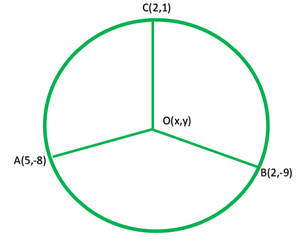Given that O is the centre of the circle and A(5, -8), B(2, -9), and C (2, 1) are the points on the circle.

So, let us considered the co-ordinates of O be (x, y)

Therefore, OA = OB = OC

OA2 = OB2 = OC2

NowOA2 = (x – 5)2 + (y + 8)2

= x2 – 10x + 25 + y2 + 16y + 64

= x2 + y2 – 10x + 16y + 89

Similarly, OB2 = (x – 5)2 + (y + 9)2

= x2 + 4 – 4x + y2 + 81 + 18y

= x2 + y2 – 4x + 18y + 85

and OC2 = x2 – 4x + 4 + y2 – 2y + 1

= x2 + y2 – 4x – 2y + 5

OA2 = OB2

x2 + y2 – 10x + 16y + 89 = x2 + y2 – 4x + 18y + 85

-10x + 4x + 16y – 18y = 85 – 89

-6x – 2y = -4

3x + y = 2                         …….(i)

OB2 = OC2

x2 + y2 – 4x + 18y + 85 = x2 + y2 – 4x – 2y + 5

18y + 2y = 5 – 85

20y = -80

y = -80/10 = -4

Now substitute the value of y in eq(i), we get

3x + y = 2

3x – 4 = 2

3x = 2 + 4 = 6

x = 6/3 = 2

Hence, the co-ordinates of O are(2,-4)

### Question 55. If two opposite vertices of a square are (5, 4) and (1, -6), find the coordinates of its remaining two vertices.

Solution: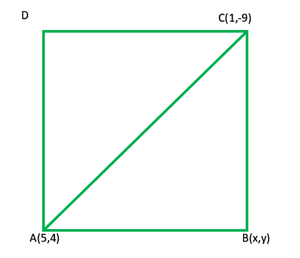Given that the two opposite points of a ABCD square are A(5, 4) and C(1, -6)

Let us considered that the co-ordinates of B be (x, y).

So, join AC

As we know that the sides of a square are equal, so,

AB = BC

AB2 = BC2

(x – 5)2 + (y – 4)2 = (x – 1)2 + (y + 6)2

x2 – 10x + 25 + y2 – 8y + 16 = x2 – 2x + 1 +y2 + 12y + 36

-10x + 2x – 8y – 12y = 37 – 41

-8x – 20y = -4

2x + 5y = 1

2x = 1 – 5y

x = (1 – 5y)/2

So, ABC is a right-angled triangle

Now by using Pythagoras theorem, we get

AC2 = AB2 + BC2

(5 – 1)2 + (4 + 6)2 = x2 – 10x + 25 + y2 – 8y + 16 + x2 – 2x + 1 + y2 + 12y + 36

(4)2 + (10)2 = 2x2 + 2y2 – 12x + 4y + 78

16 + 100 = 2x2 + 2y2 – 12x + 4y + 78

2x2 + 2y2 – 12x + 4y + 78 – 16 – 100 = 0

2x2 + 2y2 – 12x + 4y – 38 = 0

x2 + y2 – 6x + 2y – 19 = 0     …..(i)

Now substituting x = (1 – 5y)/2 in eq(i), we get1 + 25y2 – 10y + 4y2 – 12 + 60y + 8y – 76 = 0

29y2 + 58y – 87 = 0

y2 + 2y – 3 = 0

y2 + 3y – y – 3 = 0

y(y + 3) – 1(y + 3) = 0

(y + 3)(y – 1) = 0

The value of y can be either y + 3 = 0, then y = -3

or y – 1 = 0, then y = 1

When y = 1, then

x = (1 – 5y)/2

= (1 – 5(1))/2

= -2

When y = -3, then

x = (1 – 5(-3))/2

= 8

So, the other points of ABCD square are(-2,1) and (8,-3)

### Question 56. Find the centre of the circle passing through (6, -6), (3, -7), and (3, 3).

Solution: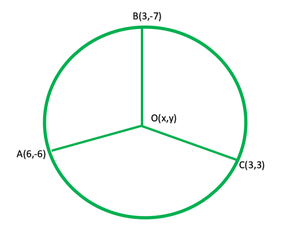Let us considered O be the centre of the circle is (x, y)

It is given that centre of the circle passing through (6, -6), (3, -7), and (3, 3)

Join OA, OB and OC

So, OA = OB = OC

OA2 = (x -6 )2 + (y + 6)

OB2 = (x – 3)2 + (y + 7)2

and OC2 = (x – 3)2 + (y-3)2

As we know that OA2 = OB2

So, (x – 6)2 + (y + 6)2 = (x – 3)2 + (y + 7)2

x2 – 12x + 36 + y2+12y + 36 = x2 – 6x + 9 + y2 + 14y + 49

x2 – 12x + 36 + y2+12y + 36 – x2 + 6x – 9 – y2 – 14y – 49 = 0

-12x + 12y + 72 + 6x – 14y – 58 = 0

-6x – 2y + 14 = 0

-6x – 2y = -14

3x + y = 7                 …..(i)

Also, OB2 = OC2

(x – 3)2 + (y + 7)2 = (x – 3)2 + (y – 3)2

x2 – 6x + 9 + y2+ 14y + 49 = x2 – 6x + 9 + y2 – 6y + 9

x2 + y2 – 6x + 58 + 14y – x2 – y2 + 6x + 6y – 18 = 0

20y + 40 = 0

20y = -40

y = -40/20 = -2

3x + (-2) = 7

3x = 7 + 2 = 9

x = 9/3 = 3

Hence, the co-ordinates of the centre are(3,-2)

### Question 57. Two opposite vertices of a square are (-1, 2) and (3, 2). Find the coordinates of the other two vertices.

Solution: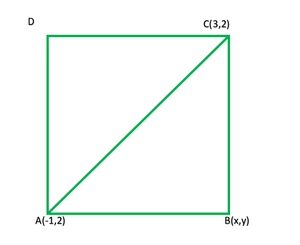Let us considered ABCD is square, in which the co-ordinates are A (-1, 2) and C (3, 2).

Let us assume the coordinates of B are (x, y)

Now, join AC

As we know that the sides of a square are equal, so,

AB = BC

AB2 = BC2

NowAB2 = (x + 1)2 + (y – 2)2

Similarly, BC2 = (x – 3)2 + (y – 2)2

As we know that AB = BC

So,

(x + 1)2 + (y – 2)2 = (x – 3)2 + (y – 2)2

(x + 1)2 = (x – 3)2

x2 + 2x + 1 = x2 – 6x + 9

x2 + 2x + 6x – x2 = 9 – 1 = 8

8x = 8

x = 8/8 = 1

Now in right triangle ABC

AC2 = AB2 + BC2

(3 + 1)2 + (2 – 2)2 = (x + 1)2 + (y – 2)2 + (x – 3)2 + (y – 2)2

(4)2 + (0)2 = x2 + 2x + 1 + y2 – 4y + 4 + x2 – 6x + 9 + y2 – 4y + 4

16 = 2x2 + 2y2 – 4x – 8y + 18

2x2 + 2y2 – 4x – 8y = 16 – 18

2x2 + 2y2 – 4x – 8y = -2

x2 + y2 – 2x – 4y = -1    …….(i)

Now substitute the value of x, in eq(i), we get

(1)2 + y2 – 2 × 1 – 4y = -1

1 + y2 – 2 – 4y = -1

y2 – 4y = -1 – 1 + 2 = 0

y(y – 4) = 0

So the value of the y can be either y = 0

or y – 4 = 0, then y = 4

Hence, the coordinates of other points will be (1, 0) and (1, 4)

My Personal Notes arrow_drop_up
Related Tutorials